PSABPQZG - Student Facing Task---Acc7.5 Lesson 25 Using Linear Relations to Solve Problems (8.EE.B.6 8.EE.C.8.a)
Part A)

For each relationship described, write an equation to represent the relationship.

Grapes cost \$2.39 per pound. Bananas cost \$0.59 per pound. You have \$15 to spend on g pounds of grapes and b pounds of bananas.

Write the equation using the "WIRIS editor" button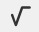Part B)

A savings account has \$50 in it at the start of the year and \$20 is deposited each week. After x weeks, there are y dollars in the account.

Write the equation using the "WIRIS editor" button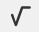Part A)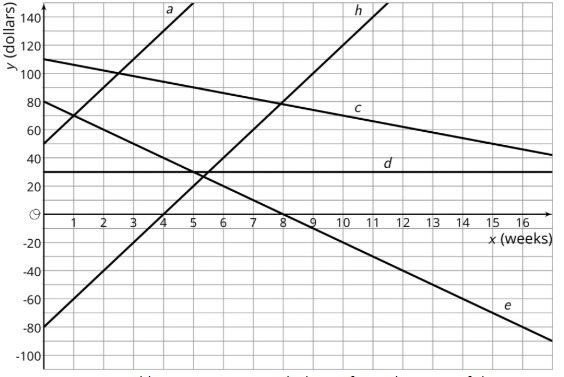Each line represents one person’s weekly savings account balance from the start of the year.

Choose one line and write a description of what happens to that person's account over the first 17 weeks of the year. Do not tell your group which line you chose.

Part B)

Write an equation for each line on the graph. What do the slope, m, and vertical intercept, b, in each equation mean in the situation?

Part C)

For which equation is (1,70) a solution? Interpret this solution in terms of your story.

Part D)

Predict the balance in each account after 20 weeks.

Person will have \$_____

Type your answer below as a number (example: 5, 3.1, 4 1/2, or 3/2):
Part E)

Predict the balance in each account after 20 weeks.

Person will have \$_____

Type your answer below as a number (example: 5, 3.1, 4 1/2, or 3/2):
Part F)

Predict the balance in each account after 20 weeks.

Person will have \$_____

Type your answer below as a number (example: 5, 3.1, 4 1/2, or 3/2):
Part G)

Predict the balance in each account after 20 weeks.

Person will have \$_____

Type your answer below as a number (example: 5, 3.1, 4 1/2, or 3/2):
Part H)

Predict the balance in each account after 20 weeks.

Person will have \$_____

Type your answer below as a number (example: 5, 3.1, 4 1/2, or 3/2):
Part A)

The Fabulous Fish Market orders tilapia, which costs \$3 per pound, and salmon, which costs \$5 per pound. The market budgets \$210 to spend on this order each day.

What are five different combinations of salmon and tilapia that the market can order?

Part B)

Define variables and write an equation representing the relationship between the amount of each fish bought and how much the market spends.

Part C)

Sketch a graph of the relationship. Label your axes.

Draw your graph on paper, take a picture, and upload it using the image upload icon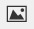If you do not have the ability to upload an image of your work, type "Graph is on paper."

Part D)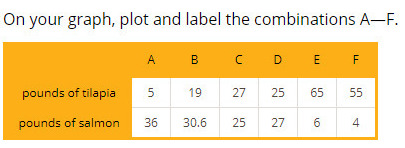Draw your graph on paper, take a picture, and upload it using the image upload iconIf you do not have the ability to upload an image of your work, type "Graph is on paper."

Part E)

Which of these combinations can the market order?

Select all that apply:
Part F)